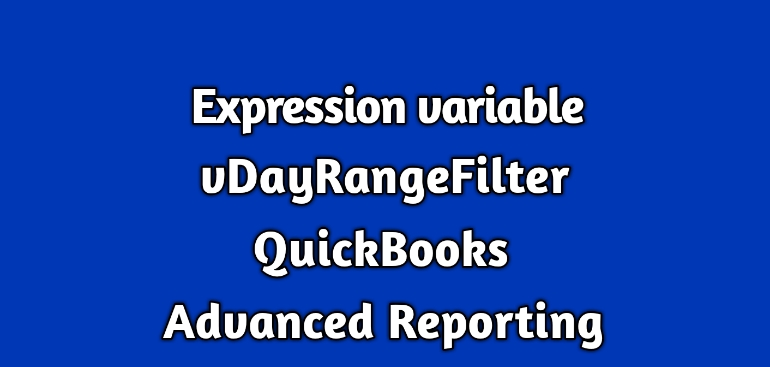# Expression variables (vDayRangeFilter) in QuickBooks Enterprise Advanced ReportingThe QuickBooks Advanced Reporting has a collection of convenient expression variables.

This blog discusses about one of them

### vDayRangeFilter:

It is defined as

[Transactions.Txn Date]={“>=\$(=Date(Max([Transactions.Txn Date])-\$1))<=\$(=Date(Max([Transactions.Txn Date])-\$2))”},Year = ,Quarter = ,Month = ,Period  = ,Week    = ,Day = ,[Period ID] =

#### Where to use it:

This expression variable is used to show amount or quantity between two different number of days.

The following expression shows sales amount and quantity between 50th and 60th days.

sum({<\$(vExprSales),\$(vDayRangeFilter(60,50))>}[Transactions.Amount With Sign])

sum({<\$(vExprSales),\$(vDayRangeFilter(60,50))>}[Transactions.Quantity With Sign])

#### Few things to note here:

• The number of days calculation is always done from the max transaction date of your company file and not from today’s date.
• The large number always goes as the first parameter to this expression.

The vDayRangeFilter is the mother of all date range-based expression variables because it can be used for any date range like monthly, quarterly, YTD or anything else.

For example,

The following expressions are for last 60 days of the current and for the same period last year respectively:

sum({<\$(vExprSales),\$(vDayRangeFilter(60,0))>}[Transactions.Amount With Sign])

Just add 365 to parameter values to get the values for the last year:

sum({<\$(vExprSales),\$(vDayRangeFilter(455,365))>}[Transactions.Amount With Sign])

Let us know if you have any questions.